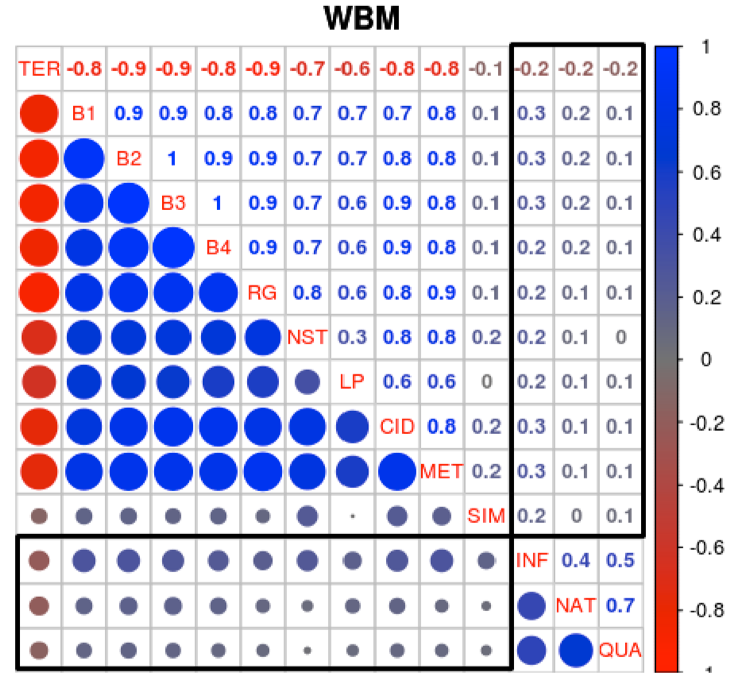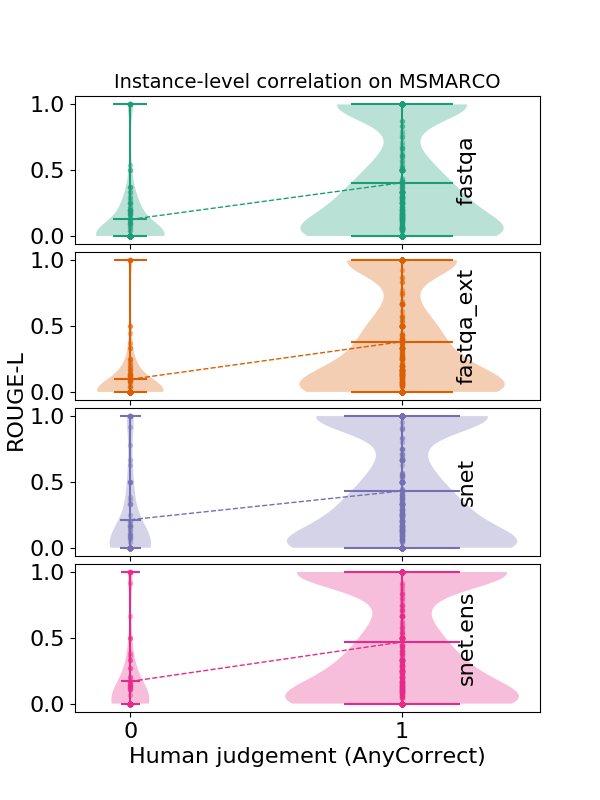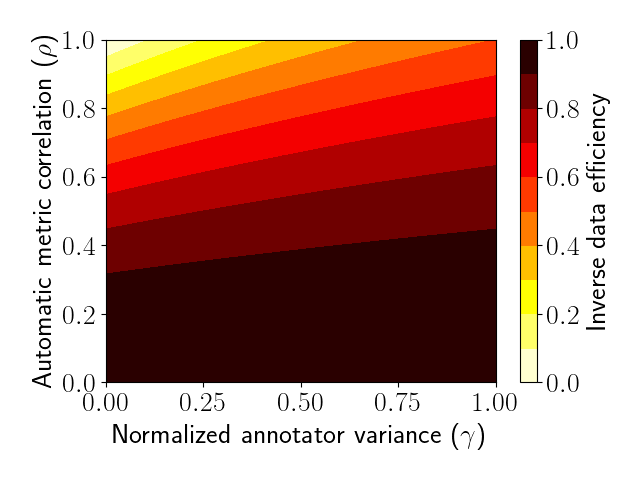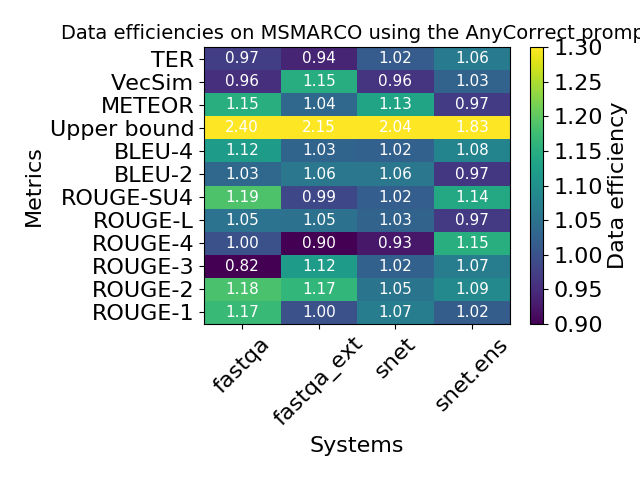# Why we need human evaluation. July 10, 2018

We’re witnessing an exciting boom in the subfield of natural language generation (NLG), with more than 150 related papers published at ACL, NAACL and EMNLP in just the last year! These papers cover a range of tasks including abstractive summarization (Nallapati et al., 2016), open-response question answering (Nguyen et al., 2016; Kočisky et al., 2017), image captioning (Lin et al., 2014), and open-domain dialogue (Lowe et al., 2017b). Unfortunately, it’s incredibly hard to compare these different methods in a meaningful way. While most papers report automatic evaluations using metrics like BLEU or ROUGE, these metrics have consistently been shown to poorly correlate with human judgment on fluency, redundancy, overall quality, etc. On the other hand, only a small fraction of these papers actually conduct a thorough human evaluation.

Is complete human evaluation really necessary? If so, what can we do to make it easier or cheaper to conduct human evaluations?

In our latest paper (appearing at ACL 2018), The price of debiasing automatic metrics in natural language evaluation, we shed light on both these questions. The TL;DR summary is:

1. existing automatic metrics are not just poor indicators of quality but are also biased (thus, at least some human evaluation is necessary) and
2. the cost of debiasing these automatic metrics is about the same as conducting a full human evaluation (automatic metrics won’t reduce costs, but we don’t yet know what will).

In this post, I’ll briefly summarize the findings in our paper and discuss what I think might be the most promising directions going forward are.

## So, what’s wrong with automatic metrics?

Our criticism with (existing) automatic metrics roughly falls into two bins. First of all, they just do not correlate well with human judgment on aspects like fluency, redundancy or overall quality. Second, and more importantly, the correlation of these automatic metrics varies significantly across systems. As a result, automatic metrics are likely to score certain systems as better than others, irrespective of their actual human evaluation scores. We consider this gap between how automatic metrics rate system responses and how humans rate them as a source of bias in our current evaluation methodology.

Automatic metrics don’t correlate with human judgment. A number of existing studies, Liu et al. (2016b) and Novikova et al. (2017) to name two recent examples, have identified poor correlations between automatic metrics and human judgment, ranging between 0 and 0.351. The figure below, reproduced from Novikova et al. (2017), is a stark illustration of the problem. The key takeaway is that all of the automatic metrics have extremely poor correlation with human judgments.Correlations between a suite of different automatic metrics (upper left square) and human evaluation (lower right square) on a dialog generation task. The automatic metrics compared are variants of BLEU (B1-B4), ROUGE (R), METEOR (MET) and vector-space similarity (SIM). The human evaluation included ratings for informativity (INF), naturalness (NAT) and overall quality (QUA). Reproduced from Novikova et al (2017)

Automatic metrics are biased. However, just looking at instance-level correlation doesn’t give us the whole picture. In principle, a poorly correlated automatic metric can still be a useful proxy to hill climb on if its errors were uniformly distributed. In practice, this is far from the case: current automatic metrics make systematic errors. In our paper, we investigate two tasks, the MS MARCO reading comprehension task and the CNN/Daily Mail (CDM) summarization task. We collected human judgments of answer correctness for MS MARCO and several measures of quality for CDM using Amazon Mechanical Turk2. The figure below visualizes the distribution of automatic metric scores for different human ratings across systems. The key takeaway is that there is a substantial set of system responses that are correct according to human judgment but are scored poorly by ROUGE-L and ROUGE-SU: put differently, ROUGE has poor recall. This finding extends to other token-based automatic metrics like BLEU or METEOR.Violinplots show the distribution of automatic metrics scores for a given human score.

Relatedly, we also observe that automatic metric correlation varies significantly across different systems: as a result, the same automatic metric score may correspond to very different human scores. The differential treatment of systems by automatic metrics is an example of bias.

Biased evaluation hinders progress. The long-term effects of bias in our evaluation methodology is complex and hard to measure. One possible consequence is that we may gradually develop systems that improve on automatic metrics without actually getting better according to human judgment. Connor and Dang (2010) find this to be case when studying how systems on the DUC summarization tasks changed from 2006 to 2008: despite still scoring poorly on human evaluation, the best automatic systems were indistinguishable from human summaries according to the automatic metric. Another possible consequence is that genuine improvements (ones that improve human judgment) may be discarded because they aren’t reflected in the automatic evaluation. Last year, we identified this to be case in the setting of knowledge base population (Chaganty et al., 2017).

## Can we debias automatic metrics?

A natural question to ask is if we can eliminate bias by augmenting our automatic metric in some way. Before continuing though, we need to define what a “correct” evaluation looks like. In our paper, we choose averaged human judgment to be our gold standard: any evaluation procedure that agrees (in expectation) with human evaluation is, by definition, unbiased. Using human evaluation as our baseline, we measure the factor reduction in the number of human annotations required when using an automatic metric in our evaluation procedure: the less annotations we need to use, the more feasible it is to debias the automatic metric. Our main result is an optimal estimator whose performance depends on just two quantities:

1. how correlated the automatic metric is with average human judgment and
2. how much variance (noise) there is in human judgments.

Unfortunately, we find that in practice debiasing automatic metrics requires almost as many human annotations as conducting a complete human evaluation.

Notation. For every possible test query, $$x \in \mathcal{X}$$, let $$S(x)$$ be the output generated by system $$S$$. Let $$Y(z)$$ be the human judgment of an input-output pair $$z = (x, S(x)) \in \mathcal{Z}$$. Note that because annotators may disagree, $$Y(z)$$ is actually a random variable. We define the ground truth $$f(z)$$ to be average human judgment: $$f(z) = \mathbb{E}[Y(z)]$$. As per our description above, unbiased evaluation corresponds to averaging over all examples, $\mu = \mathbb{E}_z[f(z)] = \frac{1}{|\mathcal{Z}|} \sum_{z \in \mathcal{Z}} f(z).$ We say that an estimator procedure $$\hat{\mu}$$ is unbiased iff $$\mathbb{E}[\hat{\mu}] = \mu$$.

Using this notation, standard human evaluation corresponds to taking the simple mean over $$n$$ human judgments: $\hat{\mu}_{\text{mean}}= \frac{1}{n} \sum_{i=1}^n y^{(i)},$ where $$y^{(i)}$$ is the human annotation received on the $$i$$-th pair $$z^{(i)}$$. It should be easy to see that $$\hat{\mu}_{\text{mean}}$$ is unbiased.

More formally, we would like to find an unbiased procedure $$\hat{\mu}$$ that can combine human annotations $$Y(z^{(1)}), \ldots, Y(z^{(n)})$$ with the predictions made by an automatic metric $$g(z)$$, $$g(z^{(1)}), \ldots, g(z^{(n)})$$ that requires fewer human annotations than $$\hat{\mu}_{\text{mean}}$$ to estimate $$\mu$$ to within a confidence interval.

Using control variates optimally integrates human annotations with an automatic metric. In our paper, we propose a method to do just that uses control variates. Fundamentally, the estimator exploits the fact that if two quantities are correlated, their difference will have less variance than the quantities on their own. We’ve illustrated this using a simple 1-d function below:Consider a gross simplification of the evaluation problem: we wish to measure the mean of the 1-d blue curve, $$f(z)$$. We can do so by randomly sampling a few values of $$z$$ and computing the average $$f(z)$$ values; the inherent variance of this approach depends only on the variance of $$f(x)$$. If we have access to another function, $$g(z)$$, the difference between $$f(z)$$ and $$g(z)$$ is guaranteed to have less variance if they are correlated. This is the key insight behind the control variates method.

Ultimately, the control variates estimator is rather simple: $\hat{\mu}_{\text{cv}}= \frac{1}{n} \sum_{i=1}^n y^{(i)} - \alpha g(z^{(i)}),$ where $$\alpha = \operatorname{Cov}(f(z), g(z))$$ is a scaling factor that prevents $$g(z)$$ from introducing noise when it is poorly correlated with $$f(z)$$.

In the paper, we prove that this estimator is actually minimax optimal, meaning that no other estimator has a better variance than $$\hat{\mu}_{\text{cv}}$$ on all possible inputs3. It’s a pretty strong result that allows us to use the performance of this estimator as an upper bound on how any other estimator might do.

Possible cost savings are fundamentally limited. In the paper, we characterize the cost of debiasing automatic metrics by measuring the number of human annotations required to estimate $$\mu$$ using the automatic metric relative to a complete human evaluation. This ratio is also equal to the ratio of variances of the two estimators and is called data efficiency. We show that the data efficiency of the control variates estimator is: $\text{DE} = \frac{\operatorname{Var}{\hat{\mu}_{\text{mean}}}}{\operatorname{Var}{\hat{\mu}_{\text{cv}}}} = \frac{1 + \gamma}{1 - \rho^2 + \gamma},$ where $$\gamma = \frac{\operatorname{Var}{Y}}{\operatorname{Var}{f}}$$ is the normalized annotator variance and $$\rho = \frac{\operatorname{Cov}(f(z), g(z))}{\sqrt{\operatorname{Var}{f(z)} \operatorname{Var}{g(z)}}}$$ is the correlation between the automatic metric and human judgment. This formula shows that the cost of debiasing an automatic metric depends only upon how noise annotations are and how good the automatic metric is. The table below uses the formula to visualize the (inverse) data efficiency for different values of $$\rho$$ an $$\gamma$$.A contour plot of inverse data efficiency for different values of annotator variance $$\gamma$$ and automatic metric correlation $$\rho$$.

In theory, it’s possible to have unbounded data efficiencies as $$\rho \to 1$$ and $$\gamma \to 0$$, but in practice, the values of $$\rho$$ and $$\gamma$$ are far from optimal. As a result, the data efficiencies are quite modest:Data efficiencies of the control-variates estimator for different systems and automatic metrics.

I’d like to stress that these low data efficiencies are not simply a result of the control variates method we’ve proposed, but rather describe fundamental limitations in debiasing automatic metrics.

## What’s next?

We’ve shown that it is hard to eliminate bias in automatic metrics without having to do an almost complete human evaluation. Our theory shows that there are two main ways to reduce the cost of debiasing automatic metrics: decreasing the annotator variance through better annotation prompts and developing better automatic metrics with higher correlations (about 0.6-0.9 is needed for any substantial gains).

As an example of developing better annotation prompts, we found that asking annotators to post-edit system generated summaries reduced annotator variance by a factor of about 3 relative to a 3-point Likert rating scale. Using post-edits as our human judgments significantly improved data efficiencies, but they are still modest due to poor metric correlation. Other directions we think might be worth exploring are introducing explicit models of annotators (Dawid and Skene, 1979; Passonneau and Carpenter, 2014).

While improving annotation prompts are necessary, the biggest bottleneck remains developing automatic metrics that actually correlate with ground truth. There have been some attempts to learn automatic metrics, e.g. Lowe et al. (2017), but so far these approaches have not generalized well to systems that are not part of the training data.

Thanks for reading! For more details, check out our paper or CodaLab worksheet. If you have any comments or questions, send me an email at chaganty@cs.stanford.edu or tweet me at [@arunchaganty](https://twitter.com/arunchaganty). Also, if you are at ACL 2018, drop by my poster on Monday between 12:30pm and 2pm in Poster Session 1E!

## Footnotes

1. You may be wondering how these results square with work from more than 10 years ago that reported much higher correlations; for example when ROUGE was introduced in Lin et al (2004), measured a correlation of 0.99 with human judgment. There are a couple of things going on here. First, the ROUGE result was used to compare content relevance which might well be approximated by n-grams. This is no longer the way we are using the metric. Additionally, most of the systems being compared with were extractive in nature.

2. It took a lot of effort to ensure that the human evaluation itself was reliable; details regarding the design iterations and quality control measures we used can be found in the paper.

3. Sometimes minimax optimality results depend on a degenerate edge case. This is not so for our result: the control variates estimator is provably optimal (lower variance than any other estimator) when all noise is Gaussian.